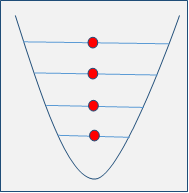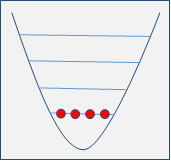# Difference Between Fermions and Bosons

## Main Difference – Fermions vs Boson

In physics, particles are classified into two groups based on their properties. They are known as fermions and bosons. Fermions are spin half particles and they obey the Pauli Exclusion Principle. But bosons are integer spin particles which do not obey the Pauli Exclusion Principle. In the standard model, fermions are the fundamental particles of matter. Bosons, on the other hand, are considered to be the force carriers. Nuclei having an odd number of nucleons are composite fermions whereas nuclei having an even number of nucleons are composite bosons. Properties of fermions and bosons are very different especially at temperatures close to the absolute zero. This article mainly focuses on the difference between fermions and bosons.

## What are Fermions

Fermions are half- integer particles and described by the Fermi-Dirac statistics. They obey the Pauli Exclusion Principle. So, two identical fermions do not occupy the same quantum state simultaneously.

Basically, fermions can be classified into two groups: elementary and composite fermions. Elementary fermions are leptons (electron, electron neutrino, muon, muon neutrino, tau, and tau neutrino) and quarks (up, down, top, bottom, strange and charm). Hadrons (neutrons, protons) containing an odd number of quarks, and nuclei made of an odd number of nucleons (Ex:  nuclei contain six protons and seven neutrons) are considered to be composite fermions. In addition, atoms such as He-3 (contain two protons, one neutron, and two electrons) are also composite fermions.

Elementary fermions are the fundamental building blocks of both matter and antimatter.## What are Bosons

Bosons are identical particles having zero or integer spins. Bosons can be categorized into two groups: elementary bosons and composite bosons. Unlike fermions, bosons do not obey the Pauli Exclusion Principle. In other words, any number of bosons can occupy the same quantum state. Behaviors of bosons are described by the Bose-Einstein statistics. The standard model only consists of five elementary bosons. They are namely the Higgs boson, gluon, photon, Z and  bosons. The Higgs boson have zero electric charge and zero spin is the only scalar boson. The last four bosons are known as gauge bosons or force carriers as they are responsible for fundamental interactions. The gluon is responsible for the strong interaction that appears between particles made of quarks. Photon is the most familiar gauge boson and is responsible for electromagnetic interactions. Z and  carry weak interaction. In addition, the mediating particle called graviton is responsible for the gravitational interaction. However, the standard model does not include the graviton. The fundamental interactions associated with the gauge bosons are described by the gauge theory.The spins and electric charges of elementary bosons are shown in the following table.

 Boson Spin Charge Interaction Z 1 0 Weak W–,W+ 1 -, + Weak Photon 1 0 Electromagnetic Gluon 1 0 Strong Graviton 2 0 Gravitational Higgs 0 0 Mass

The composite particles; mesons (contain one quark and one antiquark), and nuclei of even mass number (He- 4) are composite bosons. In addition, some quasi-particles such as cooper pairs and phonons are also considered to be bosons.

The behaviors or properties of bosons at low temperatures differ significantly from that of fermions. At very low temperatures, most of the bosons occupy the same quantum state. So a gas of bosons can be cooled temperatures very near to the absolute zero, where almost all the particles occupy the lowest energy state. At this stage, the kinetic energy of the gas is negligible. This physical phenomenon is known as Bose-Einstein condensation. The superfluidity of gasses of bosons is a consequence of Bose-Einstein condensation.## Difference Between Fermions and Bosons

### Spin

Fermions: Fermions have half- integer spin.

Bosons: Boons have integral spin.

### Pauli Exclusion Principle:

Fermions: Fermions obey the Pauli exclusion principle.

Bosons: Bosons do not obey Pauli exclusion principle.

### Examples:

Fermions: Examples include Quarks (charm), leptons (electron).

Bosons: Examples include H0, Graviton, photon, gluon, Z, .

### Statistics:

Fermions: Properties of fermions are described by the Fermi-Dirac statistics.

Bosons: Properties of bosons are described by the Bose-Einstein statistics.

### Electric charge of the elementary particles:

Fermions: Electron, muon, and tau are electrically charged leptons. But their neutrinos have no electric charge. Quark particles have fractional electric charges.

Bosons: Elementary bosons carry no electric charge (Except W bosons).

### Composite Nuclei:

Fermions: Fermions contain an odd number of nucleons.

Bosons: Bosons contain an even number of nucleons.